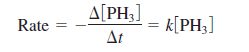Chemistry Practice Problems Identifying Reaction Order Practice Problems Solution: The rate law for the decomposition of phosphine (P...

⚠️Our tutors found the solution shown to be helpful for the problem you're searching for. We don't have the exact solution yet.

# Solution: The rate law for the decomposition of phosphine (PH 3) isIt takes 120. s for 1.00 M PH 3 to decrease to 0.250 M. How much time is required for 2.00 M PH 3 to decrease to a concentration of 0.350 M?

###### Problem

The rate law for the decomposition of phosphine (PH 3) isIt takes 120. s for 1.00 M PH 3 to decrease to 0.250 M. How much time is required for 2.00 M PH 3 to decrease to a concentration of 0.350 M?

Identifying Reaction Order

Identifying Reaction Order

#### Q. The dimerization of butadiene2C4H6 (g) → C8H12 (g)was studied at 500. K, and the following data were obtained:Assuming thatRate = - Δ [C4H6] / Δtdeter...

Solved • Mon Nov 05 2018 18:15:48 GMT-0500 (EST)

Identifying Reaction Order

#### Q. Another radioisotope of iodine, 131I, is also used to study thyroid function (see Follow-up Problem 16.7A). A patient is given a sample that is 1.7 x ...

Solved • Thu Nov 01 2018 15:05:39 GMT-0400 (EDT)

Identifying Reaction Order

#### Q. For the decomposition of gaseous dinitrogen pentoxide,2N2O5(g) ⟶ 4NO2(g) + O2(g)the rate constant is k = 2.8 x 10-3 s-1 at 60°C. The initial concentra...

Solved • Thu Nov 01 2018 14:41:55 GMT-0400 (EDT)

Identifying Reaction Order

#### Q. For the decomposition of gaseous dinitrogen pentoxide,2N2O5(g) ⟶ 4NO2(g) + O2(g)the rate constant is k = 2.8 x 10-3 s-1 at 60°C. The initial concentra...

Solved • Thu Nov 01 2018 14:41:27 GMT-0400 (EDT)